Courses

# Clausius Clapeyron Equation - Thermochemistry Chemistry Notes | EduRev

## Physical Chemistry

Created by: Asf Institute

## Chemistry : Clausius Clapeyron Equation - Thermochemistry Chemistry Notes | EduRev

The document Clausius Clapeyron Equation - Thermochemistry Chemistry Notes | EduRev is a part of the Chemistry Course Physical Chemistry.
All you need of Chemistry at this link: Chemistry

Clausirs Clapeyron Equation: The two phases in equilibrium may be any of the following types:

(i)  Solid and Liquid, S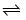L, at the melt ing point of the solid.

(ii)  Liquid and vapour, LV, at the boiling point of the liquid.

(iii)  Solid and vapour, SV; at the sublimat ion temperature of the solid. “The equal amounts of a given substance must have exactly the same free energy in the two phases at equilibrium with each other.”

Consider t ype equation AB at the given temperature and pressure. If G& GB be the free energy per mole of the substance A & B in initial.
Then, G= GB
Hence ΔG = GB - GA = 0 ΔG = GB - GA = 0
If temperature of the system raise from T to T + dT then pressure raise from P to P + dt, in order to maintain the equilibrium. The free energy of substance raise from GA to GA + dGand GB + dGB. Since the two phase are still in equilibrium hence,
CA = dGA = GB + dG                                            [∵ GA = GB]
then dGA = dGB                                          …(a)

According to thermodynamics, dG = VdP – SdT

dGB = VBdP – SBdT

Using equation (a) and (b)

we get

dGA = dGB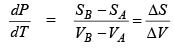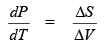V& VB be the volume of A and B.

If q heat exchanged reversibly per mo le of substance during the phase transformat ion at temperature t, then change in entropy. Δ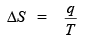Hence,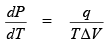Thus,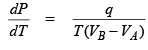This is Clapeyron equation.
Claypeyron equation for liquidvapour equilibrium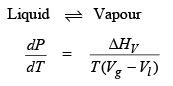where

ΔH= heat of vapourisation
Vg = volume of gas
Vl = volume of liquid
⇒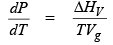[∵ Vg >> Vl]
& PV = RT (per mole)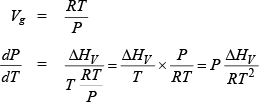⇒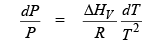⇒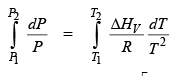⇒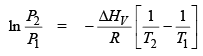⇒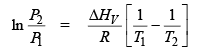⇒This is Clasusius-Clapeyron equation.
Claypeyron equation for solidvapour equilibria
SolidVapour

ΔHS = heat of sublimation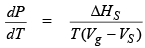Vg >> V&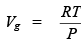Similarly the above equation, we get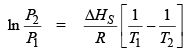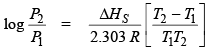Claypeyron equation for solidliquid equilibria

SolidLiquid

ΔH= heat of fusion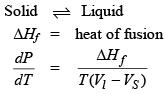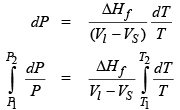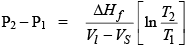Offer running on EduRev: Apply code STAYHOME200 to get INR 200 off on our premium plan EduRev Infinity!

## Physical Chemistry

77 videos|83 docs|32 tests

,

,

,

,

,

,

,

,

,

,

,

,

,

,

,

,

,

,

,

,

,

;